## 基于巴西盘试验的海冰拉伸强度研究 1)

*大连理工大学工业装备结构分析国家重点实验室, 辽宁大连 116024

**国家海洋环境预报中心国家海洋局海洋灾害预报技术研究重点实验室, 北京 100081

## EXPERIMENTAL STUDY ON SEA ICE TENSILE STRENGTH BASED ON BRAZILIAN TESTS 1)

Chen Xiaodong*, Cui Haixin, Wang Anliang**, Ji Shunying,*,2)

*State Key Laboratory of Structure Analysis of Industrial Equipment, Dalian University of Technology, Dalian 116024, Liaoning, China

Marine Design & Research Institute of China, Shanghai 200011, China

**Key Laboratory of Research on Marine Hazards Forecasting, National Marine Environmental Forecasting Center, Beijing 100081, China

 基金资助: 1)国家重点研发计划重点专项.  2018YFA0605902国家重点研发计划重点专项.  2016YFC1401505国家自然科学基金.  51639004国家自然科学基金.  41576179

Received: 2020-02-13   Accepted: 2020-02-25   Online: 2020-05-18Abstract

Keywords： granular ice ; tensile strength ; Brazilian test ; porosity ; sea ice

Chen Xiaodong, Cui Haixin, Wang Anliang, Ji Shunying. EXPERIMENTAL STUDY ON SEA ICE TENSILE STRENGTH BASED ON BRAZILIAN TESTS 1). Chinese Journal of Theoretical and Applied Mechanics[J], 2020, 52(3): 625-634 DOI:10.6052/0459-1879-20-036

## 1 巴西盘劈裂试验方法与原理

### 图1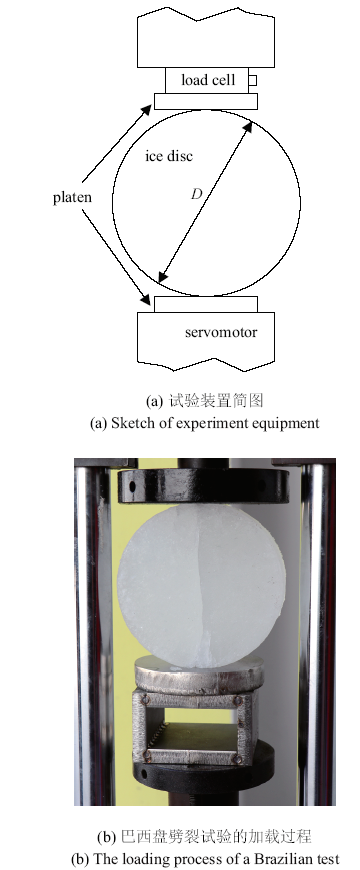Fig.1   Experimental setup of the Brazilian tests

### 图2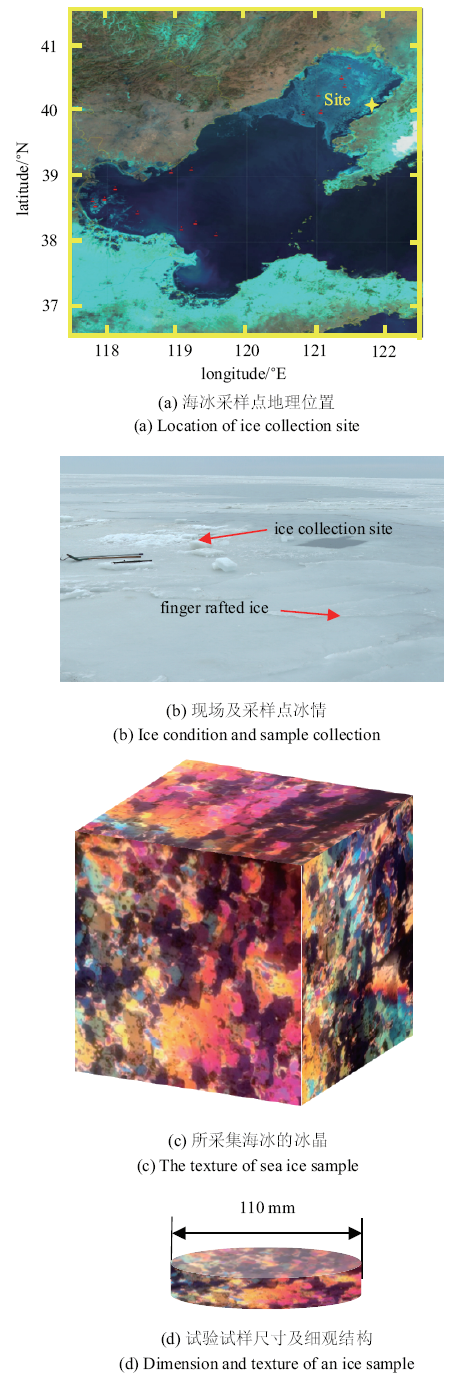Fig.2   Collection and texture of the ice sample

Table 1  The distribution of brine volume and total porosity of granular sea ice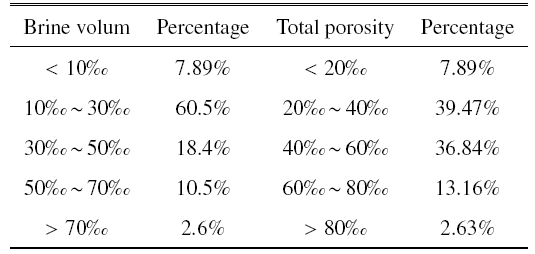### 图3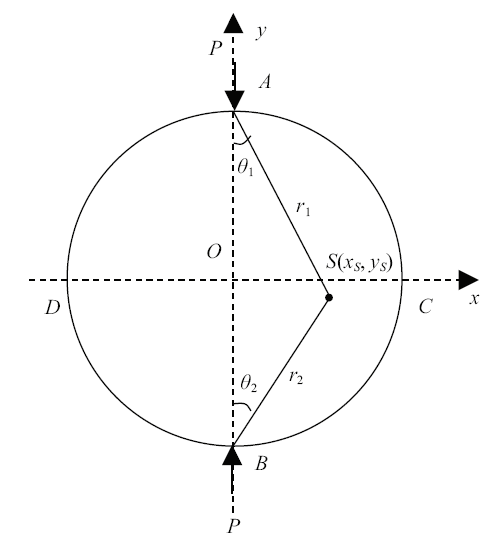Fig.3   Stress distribution within a disc under symmetrical point load

$\sigma _x =\frac{2P}{\pi L}\left( {\frac{\sin ^2\theta _1 \cos \theta _1 }{r_1 }+\frac{\sin ^2\theta _2 \cos \theta _2 }{r_2 }} \right)-\frac{2P}{\pi DL}$
$\sigma _y =\frac{2P}{\pi L}\left( {\frac{\cos ^3\theta _1 }{r_1 }+\frac{\cos ^3\theta _2 }{r_2 }} \right)-\frac{2P}{\pi DL}$

$\sigma _x =-\frac{2P}{\pi DL}$
$\sigma _y =\frac{2P}{\pi L}\left( {\frac{1}{r_1 }+\frac{1}{r_2 }}\right)-\frac{2P}{\pi DL}$

$\sigma _b =-\frac{2P_{\max } }{\pi DL}$

$\beta =\frac{w}{D}$
$\sigma \left( {\beta <0.16} \right)=\frac{2P_{\max } }{\pi DL}\left( {1-\beta ^2} \right)^{3/2}$

### 图4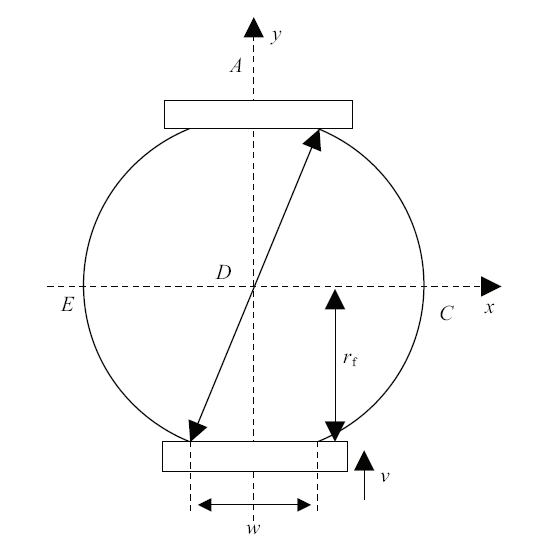Fig.4   The deformation of ice-platen contact area

$w=\sqrt {\left( {\frac{D}{2}} \right)^2-r_{f} ^2}$
$r_{f} =\frac{D-\int v {d}t}{2}$
$w=\sqrt {\left( {\frac{D}{2}} \right)^2-\left( {\frac{D-\int {v{d}t} }{2}} \right)^2}$

## 2 巴西盘劈裂试验结果与分析

### 图5Fig.5   The failure mode of sample from the Brazilian tests

### 图6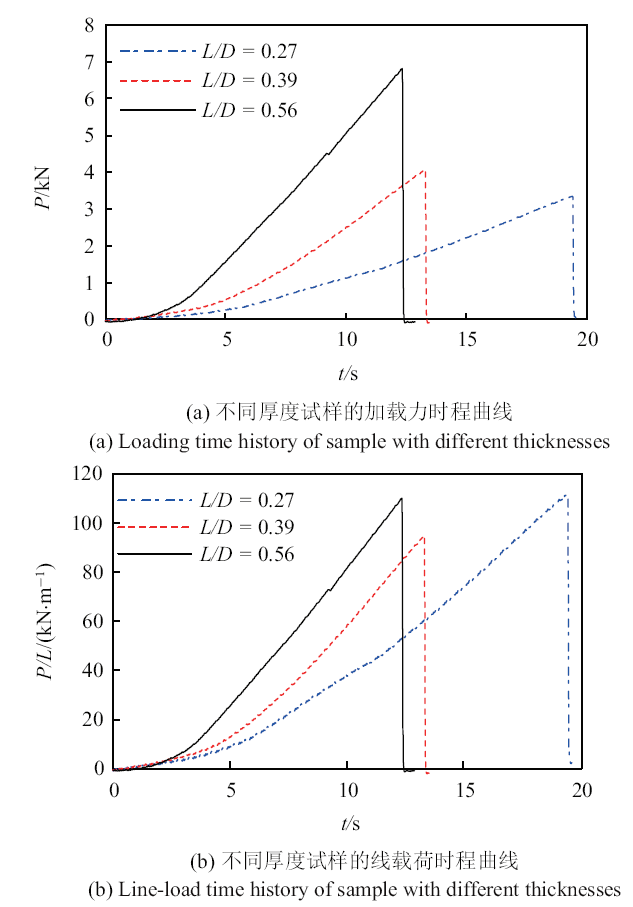Fig.6   The influence of sample thickness on the result

### 图7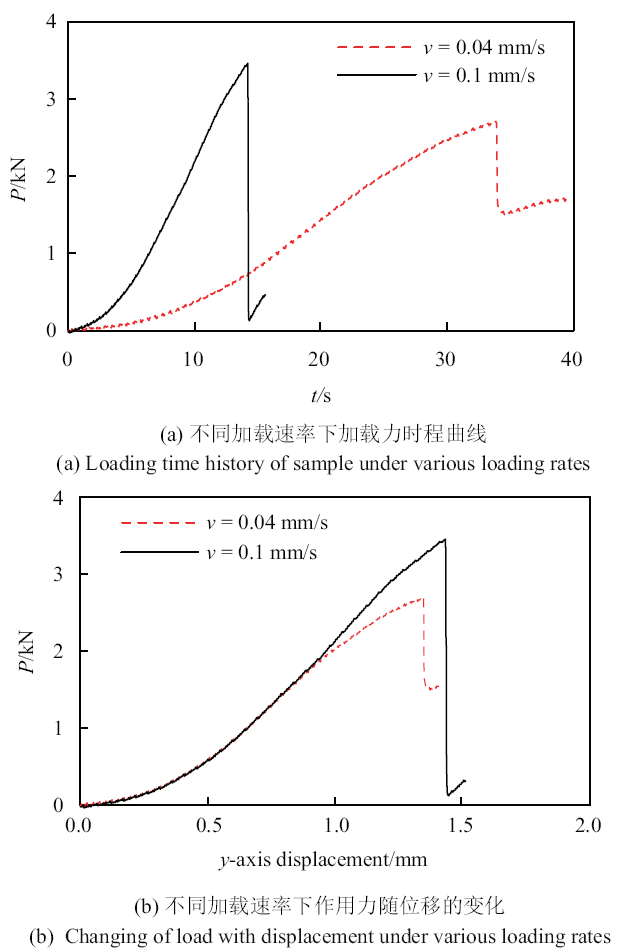### 图8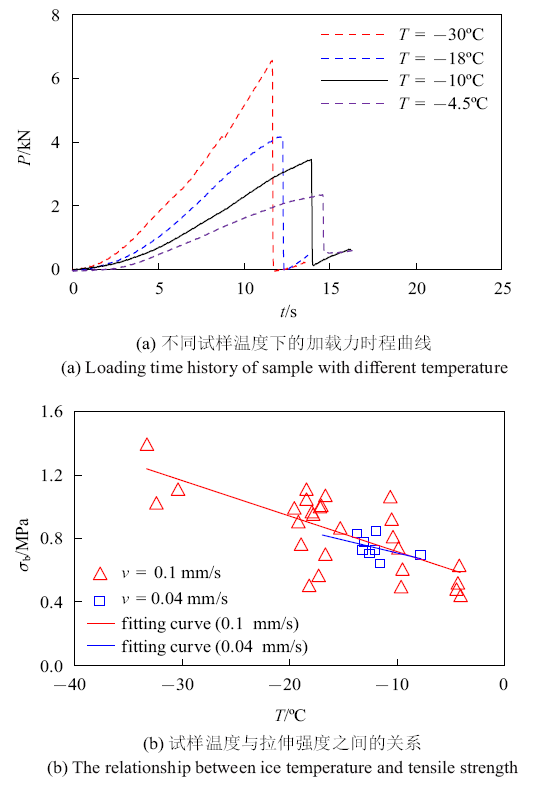Fig.8   The influence of sample temperature on the results

### 图9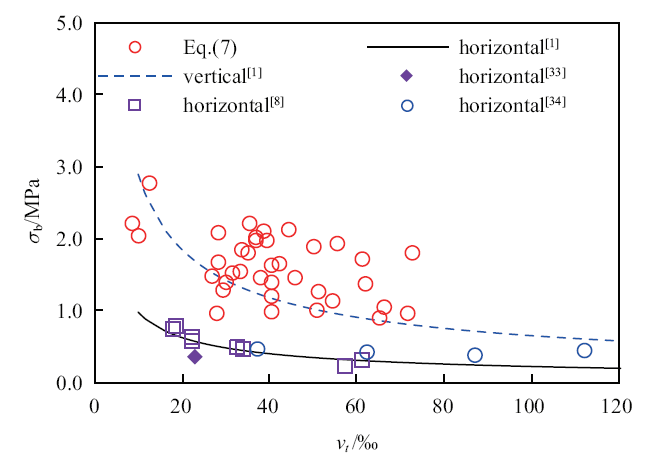Fig.9   The relationship between tensile strength and total porosity[1,8,33-34]

## 3 结论

/

 〈〉